# Mirrors and LensesPage 6

#### WATCH ALL SLIDES

p will be negative

Slide 44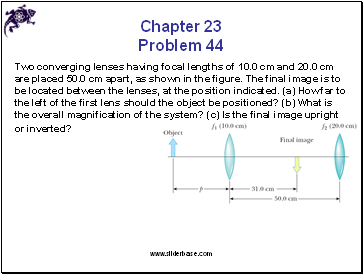Chapter 23 Problem 44

Two converging lenses having focal lengths of 10.0 cm and 20.0 cm are placed 50.0 cm apart, as shown in the figure. The final image is to be located between the lenses, at the position indicated. (a) How far to the left of the first lens should the object be positioned? (b) What is the overall magnification of the system? (c) Is the final image upright or inverted?

Slide 45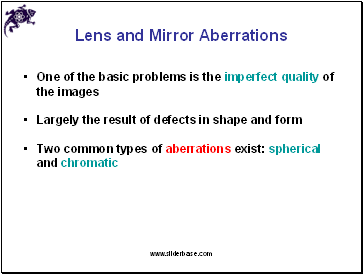## Lens and Mirror Aberrations

One of the basic problems is the imperfect quality of the images

Largely the result of defects in shape and form

Two common types of aberrations exist: spherical and chromatic

Slide 46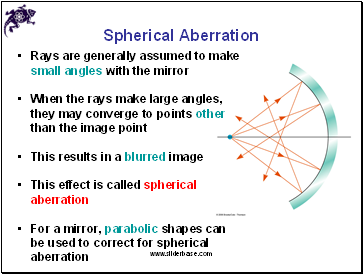## Spherical Aberration

Rays are generally assumed to make small angles with the mirror

When the rays make large angles, they may converge to points other than the image point

This results in a blurred image

This effect is called spherical aberration

For a mirror, parabolic shapes can be used to correct for spherical aberration

Slide 47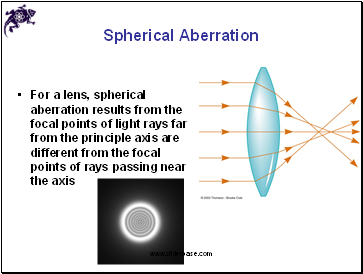Spherical Aberration

For a lens, spherical aberration results from the focal points of light rays far from the principle axis are different from the focal points of rays passing near the axis

Slide 48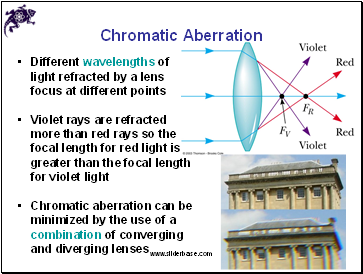## Chromatic Aberration

Different wavelengths of light refracted by a lens focus at different points

Violet rays are refracted more than red rays so the focal length for red light is greater than the focal length for violet light

Chromatic aberration can be minimized by the use of a combination of converging and diverging lenses

Slide 49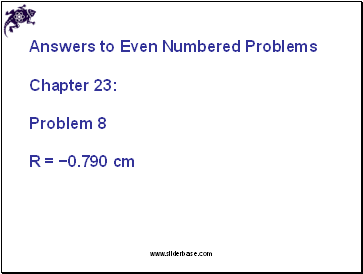Chapter 23:

Problem 8

R = −0.790 cm

Slide 50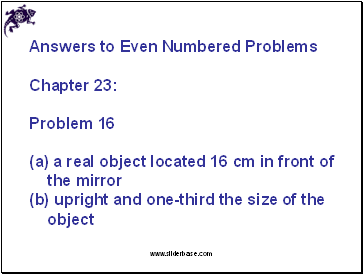Chapter 23:

Problem 16

(a) a real object located 16 cm in front of the mirror

(b) upright and one-third the size of the object

Slide 51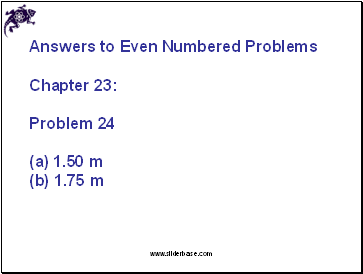Chapter 23:

Problem 24

1.50 m

(b) 1.75 m

Slide 52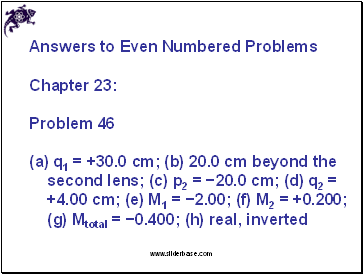Chapter 23:

Problem 46

(a) q1 = +30.0 cm; (b) 20.0 cm beyond the second lens; (c) p2 = −20.0 cm; (d) q2 = +4.00 cm; (e) M1 = −2.00; (f) M2 = +0.200; (g) Mtotal = −0.400; (h) real, inverted

Go to page:
1  2  3  4  5  6  7